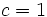# Lazard Lie group

This article defines a group property: a property that can be evaluated to true/false for any given group, invariant under isomorphism
View a complete list of group properties
VIEW RELATED: Group property implications | Group property non-implications |Group metaproperty satisfactions | Group metaproperty dissatisfactions | Group property satisfactions | Group property dissatisfactions

BEWARE! This term is nonstandard and is being used locally within the wiki. [SHOW MORE]

## Definition

### Quick definition

A group is termed a Lazard Lie group if its 3-local nilpotency class is finite and less than or equal to the group's powering threshold.

### Explicit definition

A group$G$ is termed a class$c$ Lazard Lie group for some natural number$c$ if both the following hold:

No. Shorthand for property Explanation
1 The powering threshold for$G$ is at least$c$, i.e.,$G$ is powered for the set of all primes less than or equal to$c$.$G$ is uniquely$p$-divisible for all primes$p \le c$. In other words, if$p \le c$ is a prime and$g \in G$, there is a unique value$h \in G$ satisfying$h^p = g$.
2 The 3-local nilpotency class of$G$ is at most$c$. For any three elements of$G$, the subgroup of$G$ generated by these three elements is a nilpotent group of nilpotency class at most$c$.

Condition (1) gets more demanding (i.e., stronger, so satisfied by fewer groups) as$c$ increases, while condition (2) gets less demanding (i.e., weaker, so satisfied by more groups) as we increase$c$. Thus, a particular value of$c$ may work for a group but larger and smaller values may not.

A group is termed a Lazard Lie group if it is a class$c$ Lazard Lie group for some natural number$c$.

A Lazard Lie group is a group that can participate on the group side of the Lazard correspondence. The Lie ring on the other side is its Lazard Lie ring.

### Set of possible values$c$ for which a group is a class$c$ Lazard Lie group

A group is a Lazard Lie group if and only if its 3-local nilpotency class is less than or equal to its powering threshold. The set of permissible$c$ values for which the group is a class$c$ Lazard Lie group is the set of$c$ satisfying:

3-local nilpotency class$\le c \le$ powering threshold

### p-group version

A p-group is termed a Lazard Lie group if its 3-local nilpotency class is at most$p - 1$. In other words, every subgroup of it generated by at most three elements has nilpotency class at most$p - 1$ where$p$ is the prime associated with the group.

## Metaproperties

Metaproperty name Satisfied? Proof Statement with symbols
subgroup-closed group property No Lazard Lie property is not subgroup-closed It is possible to have a Lazard Lie group$G$ and a subgroup$H$ of$G$ such that$H$ is not a Lazard Lie group in its own right.
quotient-closed group property No Lazard Lie property is not quotient-closed It is possible to have a Lazard Lie group$G$ and a normal subgroup$H$ of$G$ such that the quotient group$G/H$ is not a Lazard Lie group in its own right.
finite direct product-closed group property No Lazard Lie property is not finite direct product-closed It is possible to have Lazard Lie groups$G_1$ and$G_2$ such that the external direct product$G_1 \times G_2$ is not a Lazard Lie group.

## Relation with other properties

### Stronger properties

Property Meaning Proof of implication Proof of strictness (reverse implication failure) Intermediate notions
abelian group any two elements commute Precisely the case$c = 1$ (see also Lazard correspondence#Particular cases) |FULL LIST, MORE INFO
Baer Lie group uniquely 2-divisible and class at most two Precisely the case$c = 2$ (see also Lazard correspondence#Particular cases Global Lazard Lie group|FULL LIST, MORE INFO
p-group of nilpotency class less than p global nilpotency class puts an upper bound on the 3-local nilpotency class Global Lazard Lie group|FULL LIST, MORE INFO
rationally powered nilpotent group nilpotent and uniquely divisible for all primes Global Lazard Lie group|FULL LIST, MORE INFO